# Heat Exchanger : Types and Performance

Sharing is Caring :)-

Heat Exchangers are devices in which heat is transferred between two fluids at different temperature without any mixing of the fluids. Some specific applications of heat exchangers are space heating, air conditioning, power Production, Waste Heat recovery and chemical processing etc.  Today, we will discuss about heat exchangers its Types and its Performance.

# Types of Heat Exchanger:

• Direct Transfer Type Heat Exchangers: In this type of heat exchangers, cold and hot fluids flow simultaneously through device and heat is transferred through a wall separating the fluids.
• Storage Type Heat Exchangers: In Storage type of heat transfer from hot fluid to cold fluid occurs through a coupling medium in form of a porous solid matrix. The hot and cold fluids flow alternatively through matrix, the hot fluid stores heat in it and cold fluid extracts heat from matrix.
• Direct Contact Type Heat Exchangers: This is one in which the two fluids are not separated. If heat is to be transferred between gas and a liquid, the gas is either bubbled through the liquid or the liquid is sprayed in form of droplets into gas.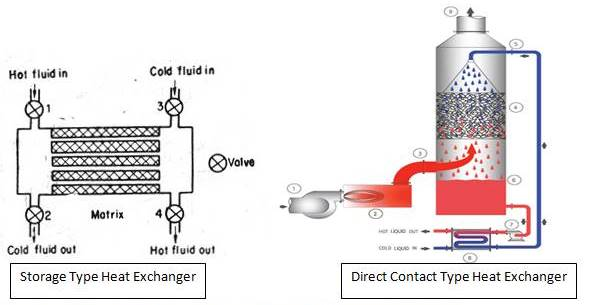## Classification of Heat exchanger based on flow arrangement:

• Parallel Flow Exchanger: In parallel flow Heat Exchanger, Hot and cold fluid enters at same end, flow in same direction and leave at same end.
• Counter flow Exchanger: In counter flow, fluids enter at opposite end, flow in opposite direction and leave at opposite direction.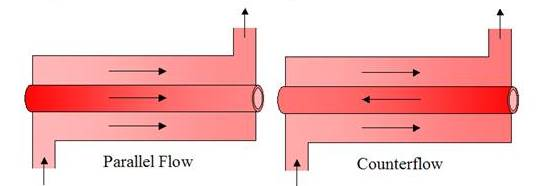• Cross flow Exchanger: In cross flow, two fluids flow in directions normal to each other. Cross flow is further classified into unmixed or mixed depending upon flow. In figure 1, cross flow is mixed as now fluid is free from moving in transverse direction(direction parallel to the tubes) In figure 2, cross flow is unmixed as plate fins force the fluid to flow through a particular interfin spacing and prevent it from moving it in direction to parallel to tubes. Since tube flow is unmixed, Thus, both fluids are unmixed in finned exchanger while one fluid is mixed other is unmixed in unfinned exchanger. Presence of mixing has significant impact on heat transfer.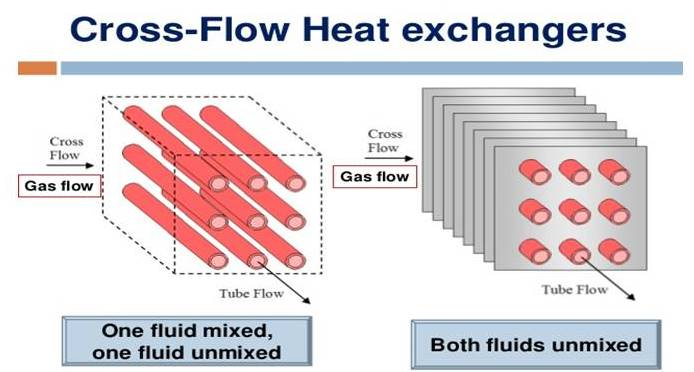## Classification according to construction:

• Shell-and-Tube Heat Exchanger: It Contains a large number of tubes packed in a shell. Heat transfer takes place as one fluid flows inside the tubes and another fluid flows outside the tube and inside the shells. Baffles are usually installed to increase convection coefficient of shell side fluid by inducing turbulence and a cross flow velocity component. They also support the tubes and reduce flow induced tube vibration.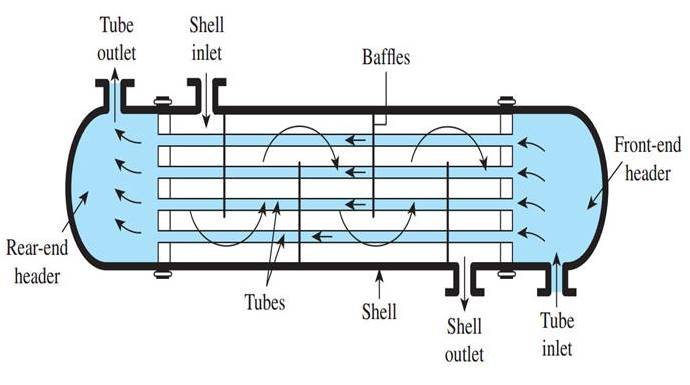• Plate Type Heat Exchanger: It has Series of large rectangular thin metal plates which are clamped together to form narrow parallel plate channel. Hot and cold fluids flow in alternate passage. These are very suitable for liquid to liquid heat exchange applications.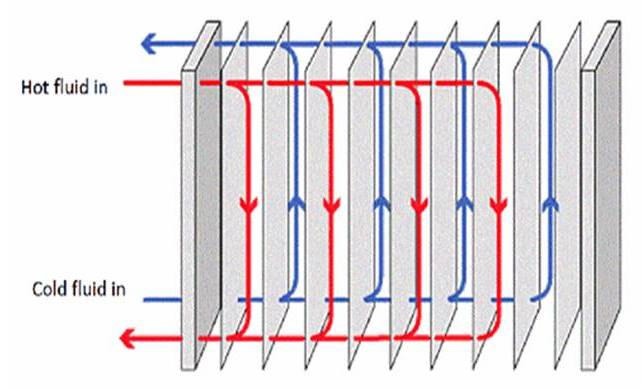• Compact Heat Exchanger: These heat exchangers have dense array of finned tube or plates and are typically used when one fluid is gas. Thus they have small convection coefficient. Tubes may be flat or circular and fins may be flat or circular.# Overall Heat Transfer Coefficient and Fouling Factor:

Overall Heat Transfer Coefficient is defined in terms of total thermal resistance to heat transfer between two fluids.

During normal Heat exchanger operation, surfaces are often subject to fouling by fluid impurities, rust formation or other reactions between the fluid and the wall material. The subsequent deposition of film or scale on the surface can greatly increase the resistance to heat transfer between the fluids. This effect can be treated by introducing a thermal resistance known as fouling factor. Its value depends upon operating temperature, fluid velocity and length of service of heat exchanger.

Thus, for a new heat exchanger fouling factor is zero and its value increases with time as solid deposits on heat exchanger surface.

### Heat Exchanger Analysis:

To predict the performance of Heat exchanger we relate the heat transfer to inlet and outlet temperature of fluids, the overall heat transfer coefficient (U) and total surface area for Heat Transfer (A).

### Energy Analysis of Heat Exchangers:

Hot fluid having inlet temperature Th,I and outlet temperature Th,o having specific heat cp,h and cold fluid having inlet temperature Tc,i and outlet temperature Tc,o having specific heat cp,c. Hot fluid flows with m ̇h and cold fluid with m ̇c.

If q is total heat transfer between hot and cold fluids and negligible heat loss between surrounding and heat exchanger, also neglecting kinetic and potential losses: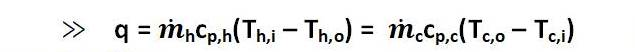This is energy equation.

### LMTD (log mean temperature difference):

We can write Heat Transfer q as: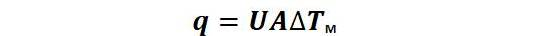Where ∆TM is appropriate mean temperature Difference. To perform a Heat Exchanger Analysis ∆TM should be known.

#### For Parallel Heat Exchangers:

Taking some assumption as

• Heat Exchanger is insulated from surrounding so that heat is exchanged between cold and hot fluid only.
• Neglecting axial conduction
• Neglecting kinetic and potential Energy
• Assuming specific Heat and U constant

We can write: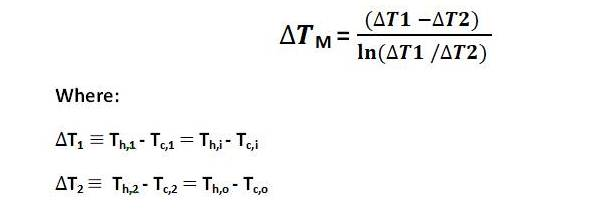#### For Counter Flow Heat Exchangers:

For Counter flow Heat Exchanger also previous equation can be used, only end point temperatures are different.We can note for same inlet and outlet temperature LMTD for counter flow exceed that for parallel flow and hence for same q and U area for counter flow is smaller than parallel flow Heat Exchangers.

Remark:

Use of LMTD Method for Heat Exchanger analysis is simple when inlet and outlet temperatures are known otherwise NTU method is preferred¬ which we will discuss in next part.

#### LMTD Using Temperature Profile:

We can easily get LMTD with temperature profile using formula given below:.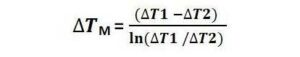For Parallel flow Heat Exchanger: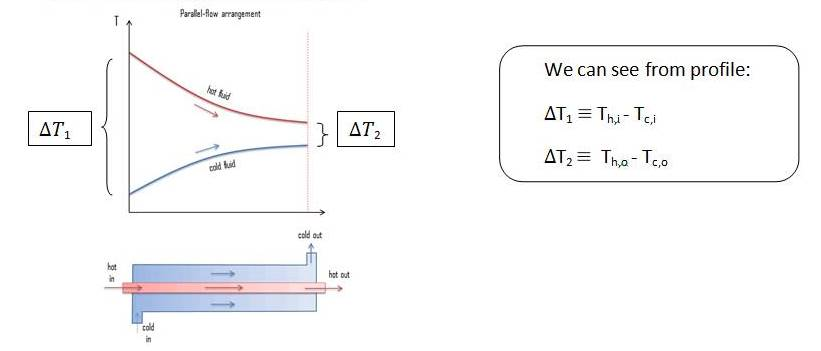For Counter Flow Heat Exchanger: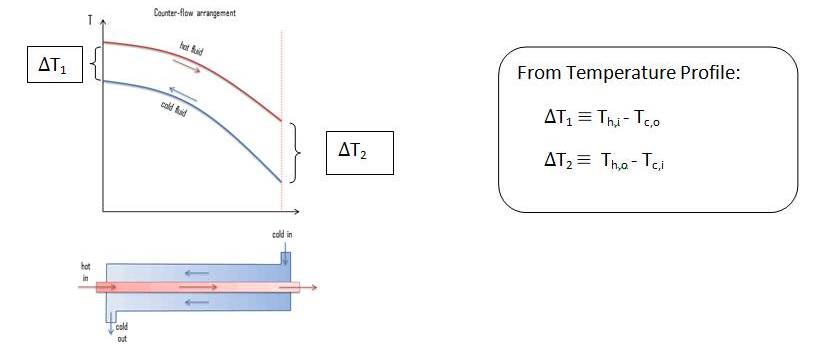Remarks:

For Counter flow Heat Exchanger with equivalent Heat capacity (Ch = Cc ):

≫ ∆T1 = ∆T2 = ∆Tm

This is all about heat exchangers and its types. If you have any query, ask by commenting.

Sharing is Caring :)-#### Abhay Porwal

This post is originally written by Abhay Porwal. He is currently studying Mechanical engineering from IIT BHU Varanasi.

### One thought on “Heat Exchanger : Types and Performance”

•October 9, 2021 at 7:14 pm